Homework Help Question & Answers

Part E - Area Under the Curve (possible 12 points) 2 Activity: In the field of statistics the fun...

i have no idea how to do this please help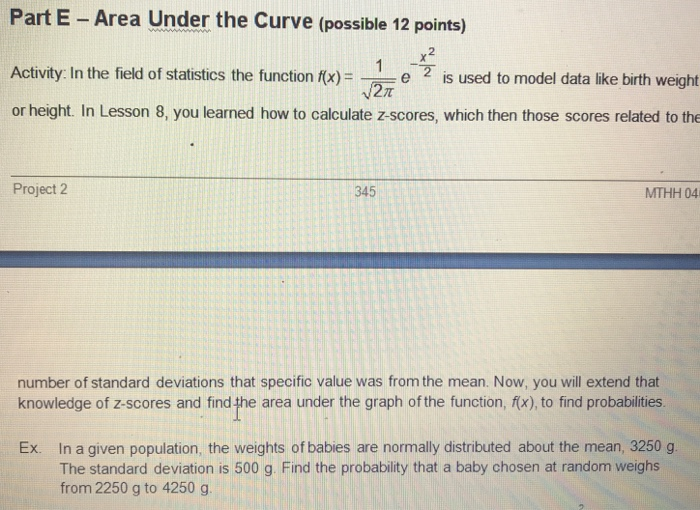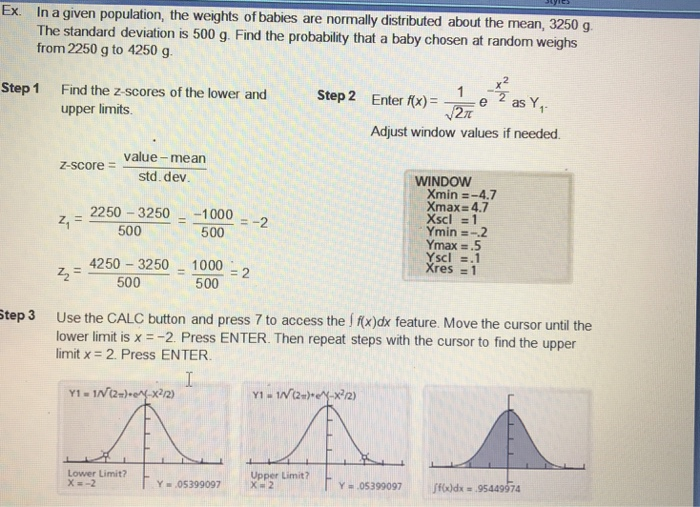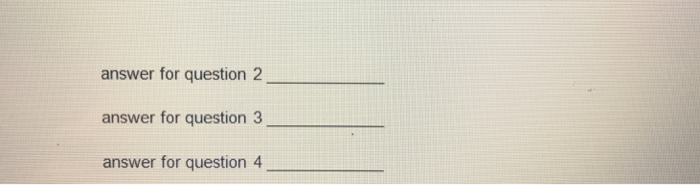Part E - Area Under the Curve (possible 12 points) 2 Activity: In the field of statistics the function fx)e 2 is used to model data like birth weight or height. In Lesson 8, you learned how to calculate z-scores, which then those scores related to the Project 2 345 MTHH 04 number of standard deviations that specific value was from the mean. Now, you will extend that knowledge of z-scores and find the area under the graph of the function, fx), to find probabilities Ex. In a given population, the weights of babies are normally distributed about the mean, 3250 g The standard deviation is 500 g. Find the probability that a baby chosen at random weighs from 2250 g to 4250g
Ex. In a given population, the weights of babies are normially distributed about the mean, 3250 g The standard deviation is 500 g. Find the probability that a baby chosen at random weighs from 2250 g to 4250 g Findthe z-scores of the lower and upper limits Step 1 Step 2 2 as Y1. Enter f(x)= e Adjust window values if needed. value-mean std. dev z-score WINDOW Xmin =-4.7 Xmax 4.7 2250-3250-10002 Ymin-.2 500 500 Ymax .5 Yscl .1 4250-3250-1000 2-500 500 Use the CALC button and press 7 to access the f #xdx feature. Move the cursor until the lower limit is x =-2 Press ENTER. Then repeat steps with the cursor to find the upper limit x=2. Press ENTER Step 3 Upper Limit? Lower Limit? Y = .05399097 ff(x)dx .95449974 Y 0539997 X 2
Upper Limit?0097 Lower Limit? x -2 UPP r Limit? Y .05399097 o)dx .95449974 Y2.05399097 The area under the curve fromx 2tox 2 is about 0.95. Therefore, the probability that a baby weighs trom 2250 g to 4250 g is about 95%. Use the data and the function in the exae in the example above. Find the probability that the weight of a baby chosen at random falls within each interval 1-4. (1 pt each) 圈 1. 3150-4150 g 2. 4300-4500 g less than 1800 g 4. more than 4550 g 3. For questions 1-4, estimate the number of babies within each interval from a population of 2400 babies. Round to nearest whole number. You will have 4 answers total. (1 pt each) 5. answer for question 1
answer for question 2 answer for question 3 answer for question 4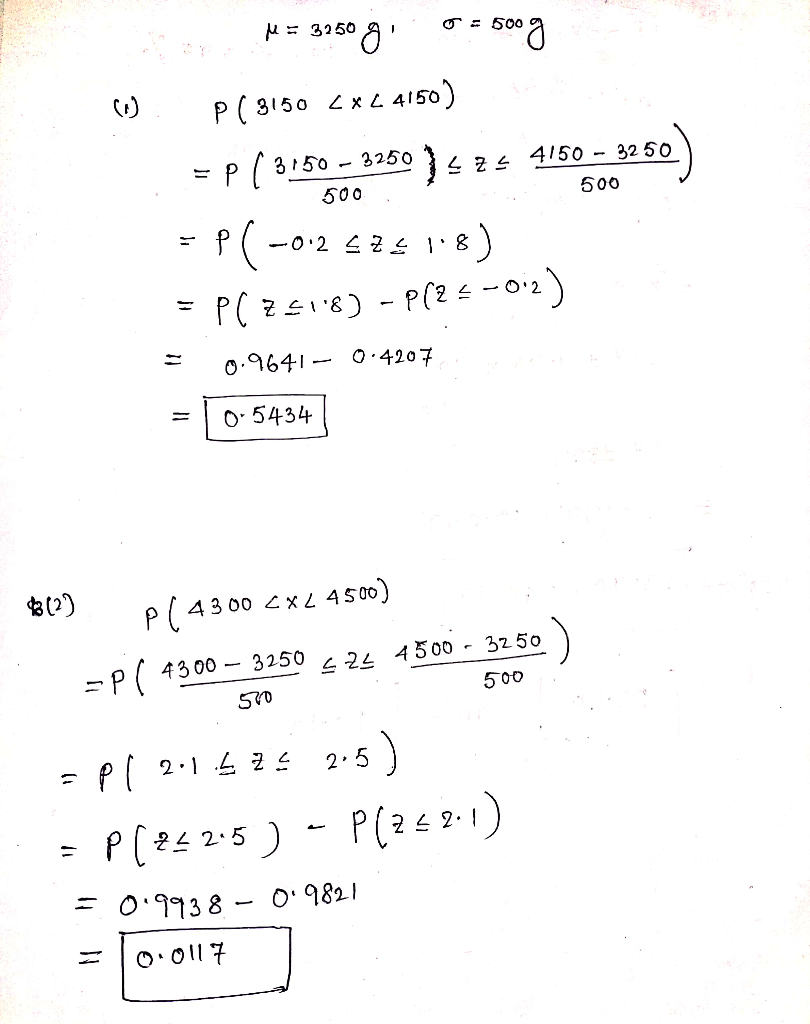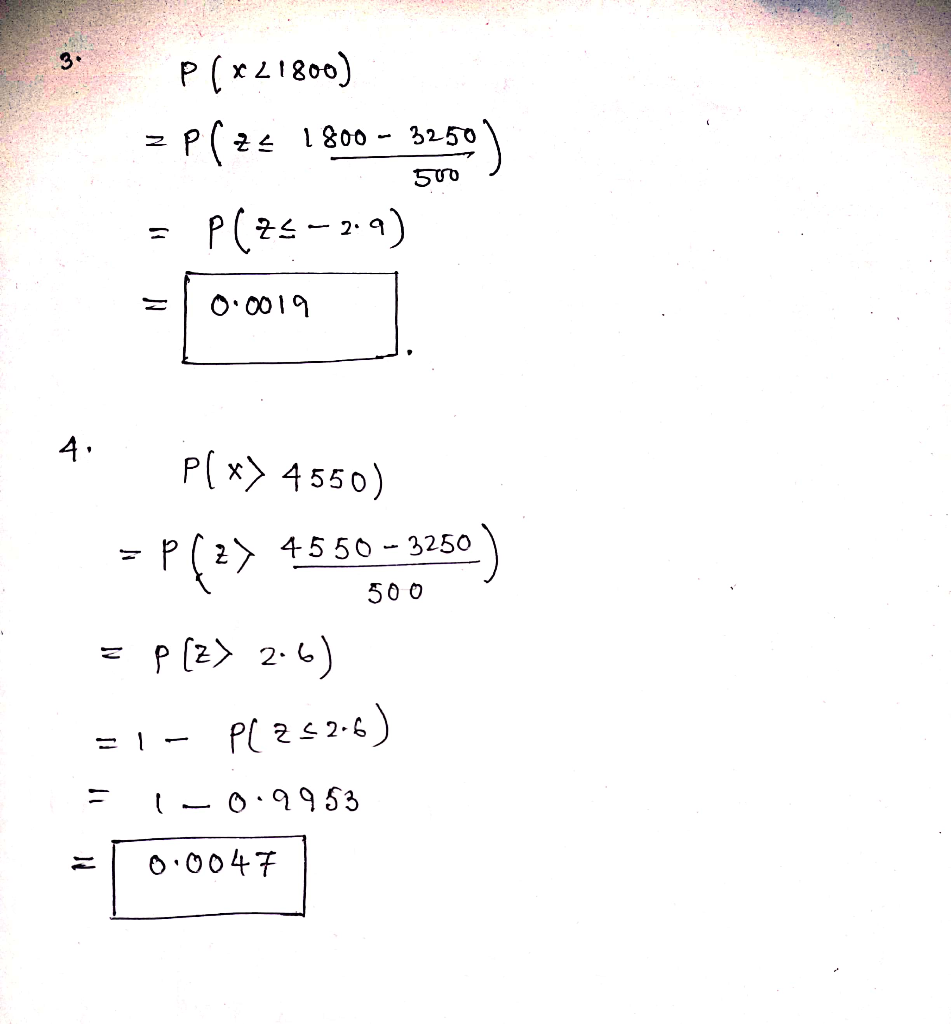Add Answer of: Part E - Area Under the Curve (possible 12 points) 2 Activity: In the field of statistics the fun...
More Homework Help Questions Additional questions in this topic.

• 5. Consider the area under the curve f(x)-on the interval [1.4), (a) Sketch the curve and identify the area of interest. (b) Approximate the area using a right-hand Riemann sum with three re...

Need Online Homework Help?

Get FREE EXPERT Answers
WITHIN MINUTES
Related Questions